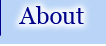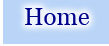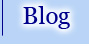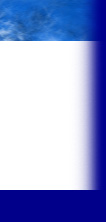﻿ tarotpathways.com » Figuring Out Your Numerological Birth Cards# Figuring Out Your Numerological Birth Cards

There are many ways to figure out birth cards in the Tarot, both numerological and astrological. The way I work with them is less complicated mathematically, and is easier for people to use than some of the more elaborate calculation systems.

To calculate numerological birth cards, do the following. We’ll use Pamela Colman Smith’s birthday of February 16, 1878 as our example.

Add Month + Day + Year:

2 + 16 + 1878 = 1896

Then, break down that number into individual digits and add again:

1 + 8 + 9 + 6 = 24

Continue breaking down the number into individual digits and adding them together until you get a number of 22 or less:

2 + 4 = 6

So Pamela’s numerological Birth card is 6, The Lovers.

If you get a number between 10 and 22, that means you have multiple numerological Birth Cards.  For example, my grandmother’s birthday initially reduces to 19, The Sun.

19 then reduces to 10 (1 + 9 = 10), so she also has the Wheel of Fortune as a birth card.

However, 10 is still a two digit number, so we reduce again: 10 is 1 + 0 = 1, and 1 is The Magician.

So my grandmother ends up with The Sun, The Wheel of Fortune, and The Magician as her numerological birth cards.  Which, I must say, suited her quite well!